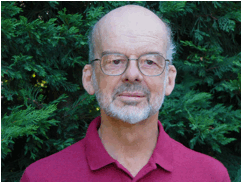# HECTOR O. FATTORINIProfessor of Mathematics (emeritus)

Research Interests: Control theory,

differential equations in linear spaces.

e-mail: hof@math.ucla.edu

Education:

Ph. D., New York University, Courant Institute of Mathematical

Sciences, 1965.

Research:

About half a century ago, the Russian mathematician L. S. Pontryagin formulated

completely for the first time the fundamental problem of the calculus of variations

and solved it with Pontryagin’s Maximum Principle. This result unified calculus of

variations and control theory of ordinary differential equations. I have been working

for many years on infinite dimensional generalizations of the maximum principle. The aim

is the control theory of partial differential equations, a subject of much theoretical and

practical interest.

Teaching:

I have worked for many years in the incorporation of technology to teaching, first using

the classical computing languages (Fortran, Pascal, C), then using computer algebra

systems (Mathematica, Maple, Matlab), e-mail and the Internet. For details see below.

The interview is a shorter version of the teaching statement.

I am presently involved in an e-textbook project together with faculty members of

several Math Departments.For details see The Live Books Project.

Books:

1. H. O. Fattorini, The Cauchy Problem, Encyclopedia of Mathematics and its

2. H. O. Fattorini Second Order Linear Differential Equations in Banach Spaces,

Notas de Matemática vol. 99, Elsevier - North Holland 1985.

3. H. O. Fattorini, Infinite Dimensional Optimization and Control Theory,

Encyclopedia of Mathematics and its Applications vol. 62, Cambridge University

Press, 1999.

4. H. O. Fattorini, Infinite Dimensional Optimization and Control Theory (reprint

edition of 3), Beijing World Publishing Company, Beijing, 2001.

5. H. O. Fattorini, Infinite Dimensional Linear Control Systems; the Time Optimal

and Norm Optimal Problems, North-Holland Mathematical Studies vol. 201,

Elsevier, Amsterdam 2005.

1. H. O. Fattorini, Sufficiency of the maximum principle for time optimality,

Cubo: A Mathematical Journal 7 (2005) 27-37.

2. H. O. Fattorini, Smoothness of the costate and the target in the time and

norm optimal problems, Optimization 55 (2006) 19-36.

3. H. O. Fattorini, Linear Control Systems in Sequence Spaces, Functional

Analysis and Evolution Equations: The Gunter Lumer Volume (2007) 273-290.

4. H. O. Fattorini, Regular and strongly regular time and norm optimal

controls, Cubo: A Mathematical Journal 10 (2008) 77-92.

5. Time and norm optimality of weakly singular controls, Progress in Nonlinear

Differential Equations and Their Applications 80 Springer Basel AG (2011)

233-249.      reprint

6. Strong regularity of time and norm optimal controls, Dynamics of Continuous,

Discrete and Impulsive Systems Series B: Applications and Algorithms 18 (2011)

436-459.      reprint                        Mathematica computations

7. Time and norm optimal controls: A survey of recent results and open

problems, Acta Matematica Scientia 31B (2011) 2203-2218.     reprint

8. Splicing of time optimal controls, Dynamic Systems and Applications 21

(2012) 169-186.